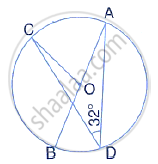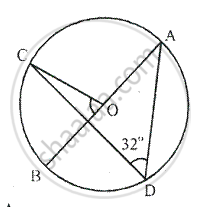Share

In the Given Figure, Ab is the Diameter of the Circle with Centre O. If ∠Adc = 32°, Find Angle Boc - ICSE Class 10 - Mathematics

ConceptArc and Chord Properties - the Angle that an Arc of a Circle Subtends at the Center is Double that Which It Subtends at Any Point on the Remaining Part of the Circle

Question

In the given figure, AB is the diameter of the circle with centre O.If ∠ADC = 32°, find angle BOC

SolutionArc AC subtends ∠AOC at the centre and ∠ADC at the remaining part Of the circle
∴ ∠AOC = 2 ∠ADC
⇒ ∠AOC = 2 × 32° = 64°
Since ∠AOC and ∠BOC are linear pair, we have
∠AOC + ∠BOC = 180°
⇒ 64° + BOC = 180°
⇒ ∠BOC = 180°
⇒ ∠BOC = 180° - 64°
⇒ ∠BOC = 116°

Is there an error in this question or solution?

Video TutorialsVIEW ALL 

Solution In the Given Figure, Ab is the Diameter of the Circle with Centre O. If ∠Adc = 32°, Find Angle Boc Concept: Arc and Chord Properties - the Angle that an Arc of a Circle Subtends at the Center is Double that Which It Subtends at Any Point on the Remaining Part of the Circle.
S